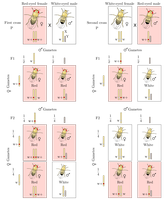# Sex-Linked Inheritance solved by 484

Dec. 7, 2012, 6:55 p.m. by Rosalind Team

Topics: Heredity, Probability

## Chromosomes Determine SexFigure 1. Morgan's two experiments on fruit fly eye color. In the first experiment, a white-eyed male is crossed with a purebred red-eyed female; in the second experiment, a red-eyed male is crossed with a white-eyed female. The results of Morgan's expermients demonstrate that eye color must be encoded by a recessive allele on the X chromosome.

In “Independent Segregation of Chromosomes”, we discussed how chromosomes in diploid organisms form pairs of homologs. It turns out that this is not the case for one pair of chromosomes in animals. In 1905, Nettie Stevens and Edmund Wilson independently discovered that male animals possess one chromosome that is shorter than its partner, whereas female animals instead have two long chromosomes. The shorter chromosome earned the title of Y chromosome for its stunted shape, whereas the longer chromosome became known as the X chromosome. These two chromosomes are aptly termed sex chromosomes, or allosomes, and we write the female sex chromosome genotype as $\textrm{XX}$ and the male genotype as $\textrm{XY}$. The remaining homologous chromosome pairs are called autosomes.

Sex chromosomes are still passed on to gametes based on the outcome of a coin flip, but egg cells (deriving from females) must always possess an X chromosome, so that the sex of an individual is determined by whether it receives an X or a Y chromosome from its father's sperm cell.

Fast-forward five years to 1910 and the lab of Thomas Hunt Morgan, who is often considered the first modern geneticist because of his tireless work to place Mendel's work on sound footing. One of Morgan's many experiments with fruit flies (genus Drosophila) began as he noticed a number of white-eyed males. When these white-eyed flies were crossed with purebred red-eyed females, their progeny were all red-eyed, and yet crossing the second generation's red-eyed individuals with each other produced some white-eyed males but exclusively red-eyed females. Strange results indeed.

Morgan's experiments are summarized in Figure 1, after which he concluded that the trait for eye color in fruit flies must be sex linked, or encoded on a sex chromosome. More specifically, the factor for white eye color is encoded by a recessive allele on the X chromosome. Because a male only has one copy of the X chromosome, having only one recessive allele will cause the individual to exhibit white eyes, whereas a female fly requires both copies of the recessive allele to possess white eyes.

X-linked recessive traits are manifested in males much more often than in females, because a male only needs to receive a recessive allele from his mother to exhibit the trait: in the case of genetic conditions, half of all male children born to carrier mothers will inherit the condition.

## Problem

The conditional probability of an event $A$ given another event $B$, written $\mathrm{Pr}(A \mid B)$, is equal to $\mathrm{Pr}(A \textrm{ and } B)$ divided by $\mathrm{Pr}(B)$.

Note that if $A$ and $B$ are independent, then $\mathrm{Pr}(A \textrm{ and } B)$ must be equal to $\mathrm{Pr}(A) \times \mathrm{Pr}(B)$, which results in $\mathrm{Pr}(A \mid B) = \mathrm{Pr}(A)$. This equation offers an intuitive view of independence: the probability of $A$, given the occurrence of event $B$, is simply the probability of $A$ (which does not depend on $B$).

In the context of sex-linked traits, genetic equilibrium requires that the alleles for a gene $k$ are uniformly distributed over the males and females of a population. In other words, the distribution of alleles is independent of sex.

Given: An array $A$ of length $n$ for which $A[k]$ represents the proportion of males in a population exhibiting the $k$-th of $n$ total recessive X-linked genes. Assume that the population is in genetic equilibrium for all $n$ genes.

Return: An array $B$ of length $n$ in which $B[k]$ equals the probability that a randomly selected female will be a carrier for the $k$-th gene.

## Sample Dataset

0.1 0.5 0.8


## Sample Output

0.18 0.5 0.32